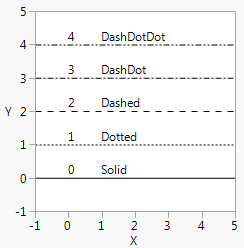Publication date: 11/10/2021

## Specify Line Types

You can also control Line Style() by number (0–4) or name (Solid, Dotted, Dashed, DashDot, DashDotDot). Figure 12.21 shows the numerical values for each line type.

`linestyles = {"Solid", "Dotted", "Dashed", "DashDot", "DashDotDot"};`
`win = New Window( "Line Styles",`
`	Graph Box(`
`		Frame Size( 200, 200 ),`
`		X Scale( -1, 5 ),`
`		Y Scale( -1, 5 ),`
`		For( i = 0, i < 5, i++,`
`			Line Style( i );`
`			H Line( i );`
`			Text( {0, i + .1}, i );`
`			Text( {1, i + .1}, linestyles[i + 1] );`
`		)`
`	)`
`);`

Figure 12.21 Line StylesTo control the thickness of lines, set a Pen Size and specify the line width in pixels. The default is 1 for single-pixel lines. For printing, think of Pen Size as a multiplier for the default line width, which varies according to your printing device.

`win = New Window( "Pen Size",`
`	Graph Box(`
`		Pen Size( 2 ); // double-width lines`
`		Line( [10 30 90], [88 22 44] );`
`	)`
`);`